# 'ggplot2' Based Publication Ready Plots

The 'ggplot2' package is excellent and flexible for elegant data visualization in R. However the default generated plots requires some formatting before we can send them for publication. Furthermore, to customize a 'ggplot', the syntax is opaque and this raises the level of difficulty for researchers with no advanced R programming skills. 'ggpubr' provides some easy-to-use functions for creating and customizing 'ggplot2'- based publication ready plots.

ggplot2, by Hadley Wickham, is an excellent and flexible package for elegant data visualization in R. However the default generated plots requires some formatting before we can send them for publication. Furthermore, to customize a ggplot, the syntax is opaque and this raises the level of difficulty for researchers with no advanced R programming skills.

The 'ggpubr' package provides some easy-to-use functions for creating and customizing 'ggplot2'- based publication ready plots.

Find out more at https://rpkgs.datanovia.com/ggpubr.

• Install from CRAN as follow:

## Distribution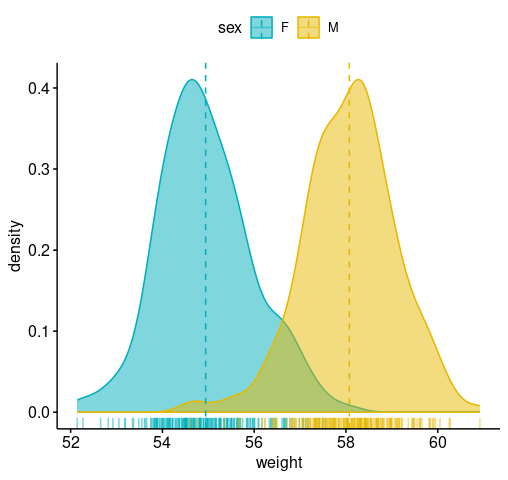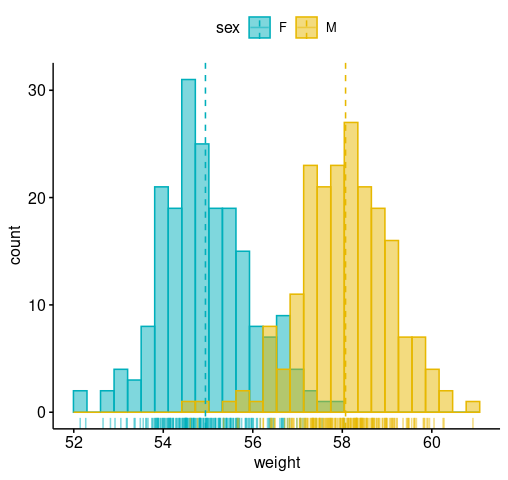## Box plots and violin plots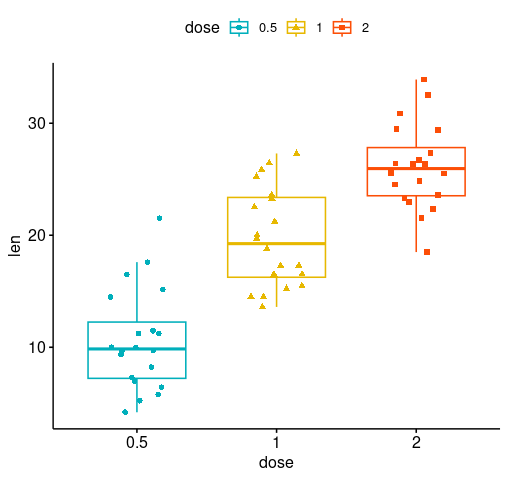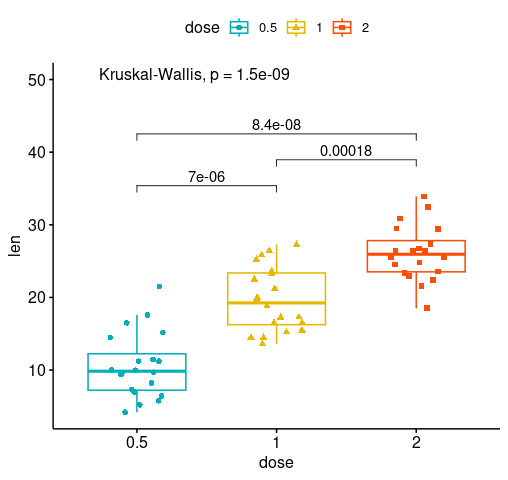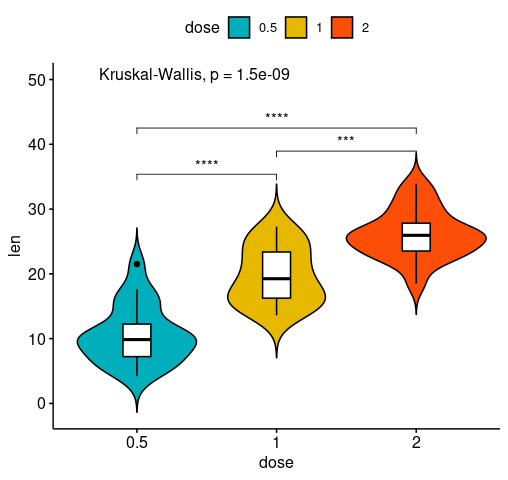## Bar plots

### Ordered bar plots

Change the fill color by the grouping variable "cyl". Sorting will be done globally, but not by groups.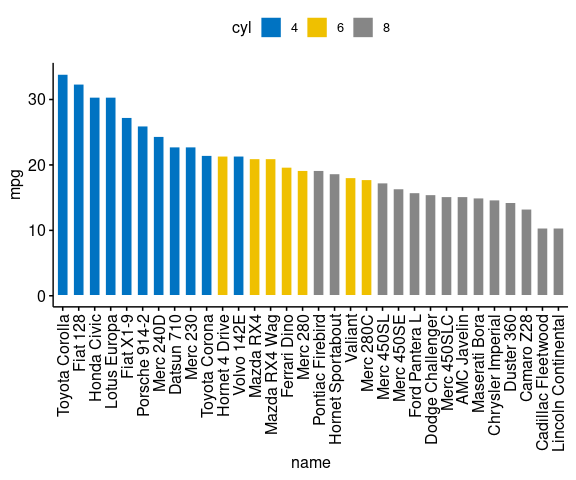Sort bars inside each group. Use the argument sort.by.groups = TRUE.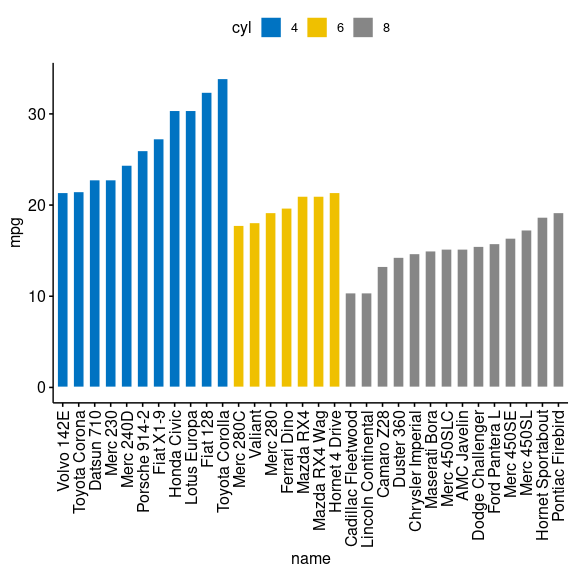### Deviation graphs

The deviation graph shows the deviation of quantitatives values to a reference value. In the R code below, we'll plot the mpg z-score from the mtcars dataset.

Calculate the z-score of the mpg data:

Create an ordered barplot, colored according to the level of mpg: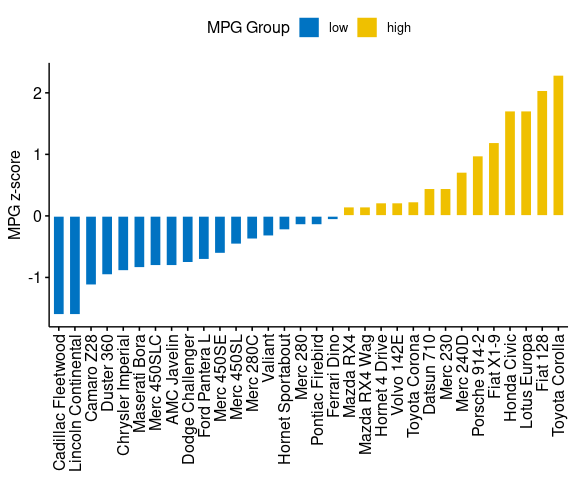Rotate the plot: use rotate = TRUE and sort.val = "desc"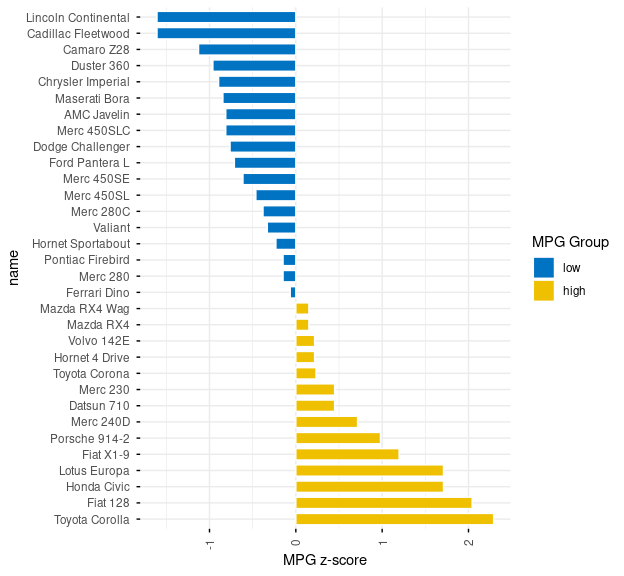## Dot charts

### Lollipop chart

Lollipop chart is an alternative to bar plots, when you have a large set of values to visualize.

Lollipop chart colored by the grouping variable "cyl":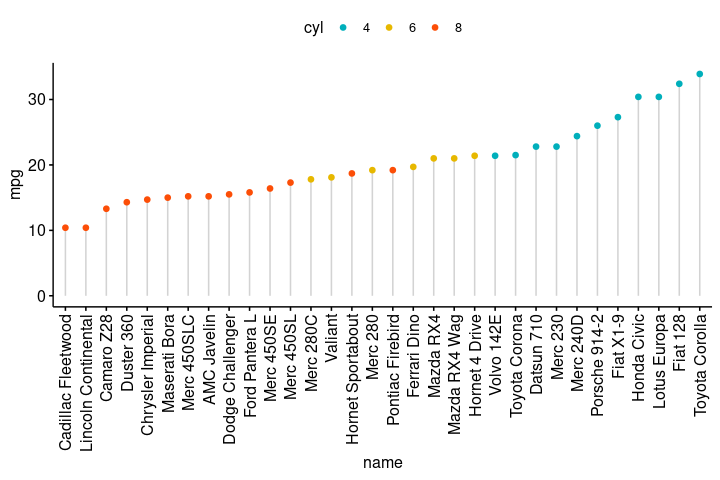• Sort in decending order. sorting = "descending".
• Rotate the plot vertically, using rotate = TRUE.
• Sort the mpg value inside each group by using group = "cyl".
• Set dot.size to 6.
• Add mpg values as label. label = "mpg" or label = round(dfm\$mpg).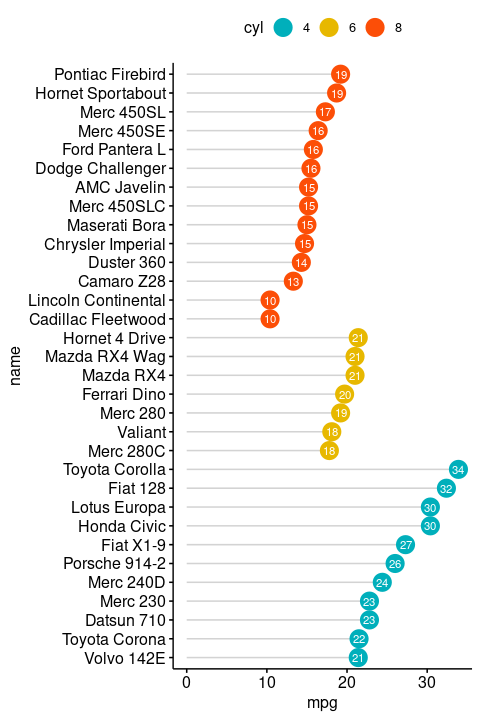Deviation graph:

• Use y = "mpg_z"
• Change segment color and size: add.params = list(color = "lightgray", size = 2)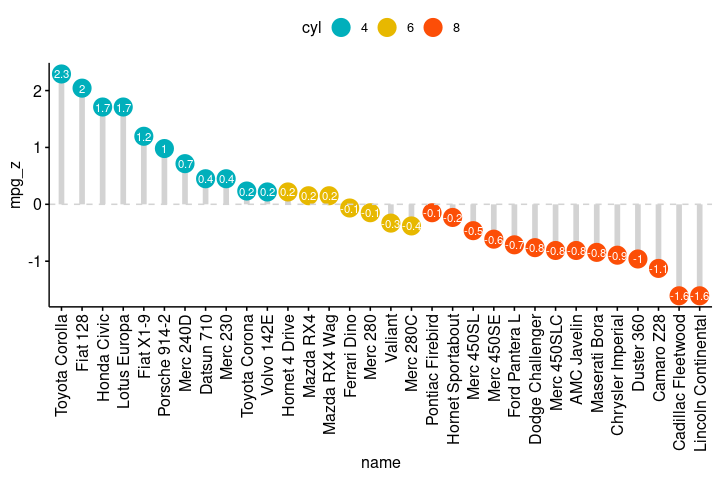### Cleveland's dot plot

Color y text by groups. Use y.text.col = TRUE.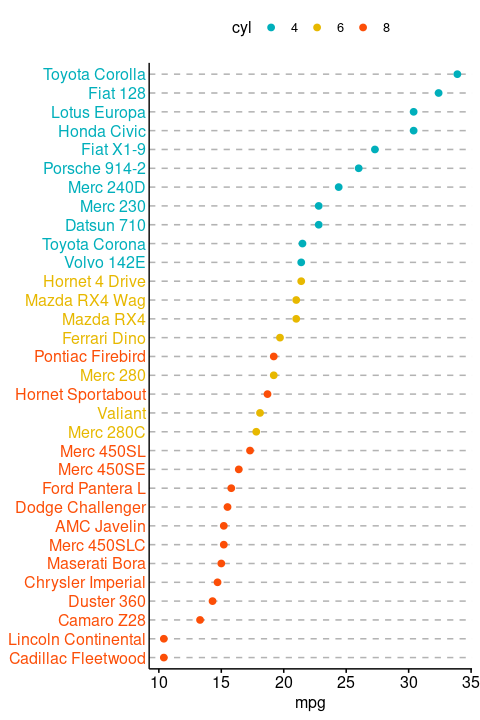## More

Find out more at https://rpkgs.datanovia.com/ggpubr.

# ggpubr 0.2

## Bug fixes

• P-value for multiple comparisons by group (stat_compare_means()) are now correctly displayed (@elisheva100, #135.

# ggpubr 0.1.9

## Minor changes

• ggsci palettes have been updated to add new palettes: nejm, jama, ucscgb, d3, locuszoom, igv, startrek, tron, futurama, simpsons (@cbrueffer, #118

## Bug fixes

• The option `ref.group` was only considered when the grouping variable contains more than two levels. In that case, each level is compared against the specified reference group. Now, `ref.group` option is also considereded in two samples mean comparisons (@OwenDonohoe, #118)

• Now, `ggqqplot()` reacts to the argument `conf.int.level` (@vsluydts, #123

• Added error bar color is now inherited from the main plot (@JesseRop, #109

# ggpubr 0.1.8

## New features

• New arguments `bxp.errorbar` added to `ggboxplot()` for adding error bars at the top of the box plots (@j3ypi, #105.
• New function `stat_pvalue_manual()` for adding p-values generated elswhere (@achamess, #81, @grst, #65).

## Minor changes

• `alpha`option added to `ggviolin()` @mtmatter, #77
• New argument `bracket.size` added to `stat_compare_means()` @mtmatter, #43
• Now, the function `stat_cor()` supports R^2 as an option @philament, #32
• New argument `position` added in `gghistogram()`. Allowed values include "identity", "stack", "dodge".
• New argument `ci` added in `ggerrorplot()` @abrar-alshaer, #94

## Bug fixes

• Now, `ggscatter()` can remove the letter 'a' from the legend, when the argument `show.legend.text = FALSE` specified @atsyplenkov, #106.
• Now, adding a `size` option to ggscatter `add.params` is supported @retrogenomics, #94.

# ggpubr 0.1.7

## New features

• New function `ggdonutchart()` added.

## Minor changes

• Significance levels can be now customized and passed to `stat_compare_means()` (@jaison75, #45).

• Editing pdf size is now supported in `ggexport()` (@JauntyJJS, #45).

## Bug fixes

• In `ggscatterhist()` the x variable was plotted two times, on both the plot x & y margins, instead of having, as expected, a) the x variable on the main plot x margin and 2) the y variable on the main plot y margin. This has been now fixed.
• In previous version, `ggdotchart()` sorted automatically within groups when the `color` argument is specified, even when groups = NULL. This default behaviour has been now removed. Sorting withi groups is performed only when the argument `group` is specified (@sfeds, #90).
• Now, `yticks.by` and `xticks.by` work with NAs (@j3ypi, #89).

# ggpubr 0.1.6

## New features

• New function `ggballoonplot()` added to visualize a contingency table.

• `ggdotchart()` can be now used to plot multiple groups with `position = position_dodge()` (@ManuelSpinola, #45).

• New function `ggscatterhist()` to create a scatter plot with marginal histograms, density plots and box plots.

• New theme `theme_pubclean()`: a clean theme without axis lines, to direct more attention to the data.

• New arguments in `ggarrange()` to customize plot labels (@G-Thomson, #41):

• font.label
• label.x and label.y
• hjust and vjust
• New argument `method.args` added to `stat_compare_means()`. A list of additional arguments used for the test method. For example one might use method.args = list(alternative = "greater") for wilcoxon test (@Nicktz, #41).

• New argument `symnum.args` added to `stat_compare_means()`. A list of arguments to pass to the function symnum for symbolic number coding of p-values. For example, `symnum.args <- list(cutpoints = c(0, 0.0001, 0.001, 0.01, 0.05, 1), symbols = c("****", "***", "**", "*", "ns"))`

• New functions `table_cell_font()` and `table_cell_bg()` to easily access and change the text font and the background of `ggtexttable()` cells (@ProbleMaker, #29).

• New argument `numeric.x.axis` in `ggline()`. logical. If TRUE, x axis will be treated as numeric. Default is FALSE. (@mdphan, #35)

• New argument `lab.nb.digits` in `ggbarplot()`. Integer indicating the number of decimal places (round) to be used (#28). Example: lab.nb.digits = 2.

• New argument `tip.length` in `stat_compare_means()`. Numeric vector with the fraction of total height that the bar goes down to indicate the precise column. Default is 0.03. Can be of same length as the number of comparisons to adjust specifically the tip lenth of each comparison. For example tip.length = c(0.01, 0.03).

## Minor changes

• Now `get_legend()` returns NULL when the plot doesn't have legend.

## Bug fixes

• Now data argument are supported in `stat_compare_means()` when the option comparisons are specified (@emcnerny, #48)

• Now `compare_means()` returns the same p-values as `stat_compare_means()` (@wydty, #15).

• `stat_compare_means()` now reacts to label = "p.format" when comparisons specified (#28).

• Now, the p.values are displayed correctly when ref.group is not the first group (@sehufnkjesktgna, #15).

# ggpubr 0.1.5

## Minor changes

• In `ggpar()`, now `legend.title` can be either a character vector, e.g.: legend.title = "Species" or a list, `legend.title = list(color = "Species", linetype = "Species", shape = "Species")`.

• New argument `ellipse.border.remove` in `ggscatter()` to remove ellipse border lines.

• In `ggscatter`(), the argument `mean.point` now reacts to fill color.

• Support for text justification added in `ggtexttable()` (@cj-wilson, #15)

• The function `ggpie()` can now display japanese texts. New argument `font.family` in `ggpie`() and in `ggpar()` (@tomochan001, #15).

• Using time on x axis works know with `ggline()` and `ggbarplot()` (@jcpsantiago, #15).

## Bug fixes

• `stat_compare_means()` now reacts to `hide.ns` properly.
• `drawDetails.splitText()` exported so that the function `ggparagraph()` works properly.
• Now, ggpubr functions accept expression for label text
• In `ggbarplot()`, now labels correspond to the true size of bars (@tdelhomme, #15).
• `stat_compare_means()` now keep the default order of factor levels (@RoKant, #12).

# ggpubr 0.1.4

## New features

• New helper functions:

• `gradient_color()` and `gradient_color()`: change gradient color and fill palettes.
• `clean_theme()`: remove axis lines, ticks, texts and titles.
• `get_legend()`: to extract the legend labels from a ggplot object.
• `as_ggplot()`: Transform the output of `gridExtra::arrangeGrob()` and `gridExtra::grid.arrange()` to a an object of class ggplot.
• `ggtexttable()`: to draw a textual table.
• `ggparagraph()`: to draw a paragraph of text.
• fill_palette() and color_palette() to change the fill and color palette, respectively.
• `annotate_figure()` to annotate (arranged) ggplots.
• `text_grob()` to create easily a customized text graphical object.
• `background_image()` to add a background image to a ggplot.
• New theme function `theme_transparent()` to create a ggplot with transparent background.

## Minor changes

• In `gghistogram()`, density curve and rug react to the fill color.
• `ggarrange()`:
• New argument `àlign` to specify whether graphs in the grid should be horizontally ("h") or vertically ("v") aligned.
• New argument `legend` to remove or specify the legend position when arranging multiple plots.
• New argument `common.legend` to create a common unique legend for multiple plots.

# ggpubr 0.1.3

## New features

• New functions:

• `ggarrange()` to arrange multiple ggplots on the same page.
• `ggexport()` to export one or multiple ggplots to a file (pdf, eps, png, jpeg).
• `ggpaired()` to plot paired data.
• `compare_means()` to compare the means of two or multiple groups. Returns a data frame.
• `stat_compare_means()` to add p-values and significance levels to plots.
• `stat_cor()` to add correlation coefficients with p-values to a scatter plot.
• `stat_stars()` to add stars to a scatter plot.
• Now, the argument `y` can be a character vector of multiple variables to plot at once. This might be useful in genomic fields to plot the gene expression levels of multiple genes at once. see `ggboxplot()`, `ggdotplot()`, `ggstripchart()`, `ggviolin()`, `ggbarplot()` and `ggline`.

• The argument `x` can be a vector of multiple variables in `gghistogram()`, `ggdensity()`, `ggecdf()` and `ggqqplot()`.

• New functions to edit ggplot graphical parameters:

• `font()` to change the appearance of titles and labels.
• `rotate_x_text()` and `rotate_y_text()` to rotate x and y axis texts.
• `rotate()` to rotate a ggplot for creating horizontal plot.
• `set_palette()` or `change_palette()` to change a ggplot color palette.
• `border()` to add/change border lines around a ggplot.
• `bgcolor()` to change ggplot panel background color.
• `rremove()` to remove a specific component from a ggplot.
• `grids()` to add grid lines.
• `xscale()` and `yscale()` to change axis scale.
• New helper functions:

• `facet()` added to create multi-panel plots (#5).
• `add_summary()` to add summary statistics.
• `ggadd()` to add summary statistics or a geometry onto a ggplot.
• New data set added: `gene_citation`

• New arguments in `ggpar()`: `x.text.angle` and `y.text.angle`

## Major changes

• New arguments in ggpubr functions, see `ggboxplot()`, `ggdotplot()`, `ggstripchart()`, `ggviolin()`, `ggbarplot()` and `ggline`:

• `combine` added to combine multiple y variables on the same graph.
• `merge` to merge multiple y variables in the same ploting area.
• `select` to select which item to display.
• `remove` to remove a specific item from a plot.
• `order` to order plot items.
• `label, font.label, label.select, repel, label.rectangle` to add and customize labels
• `facet.by, panel.labs and short.panel.labs`: support for faceting and customization of plot panels
• New argument `grouping.vars` in `ggtext()`. Grouping variables to sort the data by, when the user wants to display the top n up/down labels.

• New arguments in `theme_pubr()`:

• border,
• margin,
• legend,
• x.text.angle

## Minor changes

• Now, the argument `palette` Can be also a numeric vector of length(groups); in this case a basic color palette is created using the function `grDevices::palette()`.

# Bug fixes

• Now, `ggpar()` reacts to palette when length(palette) = 1 and palette is a color name #3.

• `ggmaplot()` now handles situations, wehre there is only upregulated, or downlegulated gnes.

# ggpubr 0.1.2

## New features

• New function `get_palette()` to generate a palette of k colors from ggsci palettes, RColorbrewer palettes and custom color palettes. Useful to extend RColorBrewer and ggsci to support more colors.

## Minor changes

• Now the `ggpar()` function can handle a list of ggplots.
• Now the default legend position is `right`.
• New argument `show.legend.text` in the `ggscatter()` function. Use show.legend.text = FALSE to hide text in the legend.
• New arguments `title, submain, subtitle, caption, font.submain, font.subtitle, font.caption` in the `ggpar()` function.
• New argument `font.family` in `ggscatter()`.

## Bug fixed

• The mean within group for `ggdensity` (`gghistogram`) are now shown if data have NA values @chunkaowang, #1

# ggpubr 0.1.1

## New features

• New function `ggtext()` for textual annotation.
• New argument star.plot in `ggscatter()`. A logical value. If TRUE, a star plot is generated.
• New helper function `geom_exec()`. A helper function used by ggpubr functions to execute any geom_xx functions in ggplot2. Useful only when you want to call a geom_xx function without carrying about the arguments to put in `ggplot2::aes()`.
• New arguments sort.val and top in `ggbarplot()`.
• sort.val: a string specifying whether the value should be sorted. Allowed values are "none" (no sorting), "asc" (for ascending) or "desc" (for descending).
• top: a numeric value specifying the number of top elements to be shown.
• New function `theme_classic2()` added. Classic theme with axis lines.

## Minor changes

• `ggboxplot()`, `ggviolin()`, `ggdotplot()`, `ggstripchart()`, `gghistogram()`, `ggdensity()`, `ggecdf()` and `ggqqplot()` can now handle one single numeric vector.
``````# Example
ggboxplot(iris\$Sepal.Length)
``````
• Now, in `gghistogram()`, when add_density = TRUE, y scale remains = "..count..".
• Now, default theme changed to theme_classic2()
• Default point size and line size set to NULL

# ggpubr 0.1.0

## Plot one variable - X: Continuous

• ggdensity(): Density plot
• gghistogram(): Histogram plot
• ggecdf(): Empirical cumulative density function
• ggqqplot(): QQ plots

## Plot two variables - X & Y: Discrete X and Continuous Y

• ggboxplot(): Box plot
• ggviolin(): Violin plot
• ggdotplot(): Dot plot
• ggstripchart(): Stripchart (jitter)
• ggbarplot(): Bar plot
• ggline(): Line plot
• ggerrorplot(): Error plot
• ggpie(): Pie chart
• ggdotchart(): Cleveland's dot plots

## Plot two continuous variables

• ggscatter(): Scatter plot

## Graphical paramters

• ggpar(): Change graphical parameters
• show_line_type(): Line types available in R
• show_point_shapes(): Point shapes available in R
• theme_pubr(): Create a publication ready theme
• labs_pubr(): Format only plot labels to a publication ready style

## Genomics

• ggmaplot(): MA-plot from means and log fold changes

## Data

• diff_express: Differential gene expression analysis results

## Other

• desc_statby(): Descriptive statistics by groups
• stat_chull(): Plot convex hull of a set of points
• stat_conf_ellipse(): Plot confidence ellipses
• stat_mean(): Draw group mean points

# Reference manual

install.packages("ggpubr")

0.2.3 by Alboukadel Kassambara, 2 months ago

https://rpkgs.datanovia.com/ggpubr/

Report a bug at https://github.com/kassambara/ggpubr/issues

Browse source code at https://github.com/cran/ggpubr

Documentation:   PDF Manual